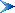World!Of
NumbersWON plate
79 |

[ November 7, 2000 ]
Some new and old discoveries about the number of the beast 666 !
Observe these hiding places of the beast in the three consecutive powers of 6n–1.

The number of the beast 666 is closely related to our unlucky number 13 !
614–1 has 6 prime factors
615–1 has 6 prime factors
616–1 has 6 prime factors

The number of the beast 666 is also the 36th triangular number!
637–1 has 6 prime factors
638–1 has 6 prime factors
639–1 has 6 prime factors
What more lies hidden e.g. behind the triplets (14,15,16) and (37,38,39) ?
Note (13+14+15)x(36+37+38) = 7 x 666 !
Lucky number 7 is playing a role as well besides the unlucky 13 !

The story isn't finished as G. L. Honaker, Jr. points out.
The 2 palindromes 666 and 555 (resulting from 666 – (36+37+38) = 555)
occur exactly once in the decimal expansion of 2666 555

3061802069160839023092406500876024752826394864138666225770884719135
20022894784390350900738050
55513810523453685782024507137361403148294
2161565170086143298589738273508330367307539078392896587187265469909Here is the first of three beautiful PALINDROMIC constructions
from Martin Gardner's book "Puzzles from other Worlds" [ Chapter 31 ]

 1 3 + 2 3 + 3 3 + 4 3 + 5 3 + 6 3 + 5 3 + 4 3 + 3 3 + 2 3 + 1 3 = 666
Secondly, the sum of the squares of the first seven prime numbers is 666.
 2 2 + 3 2 + 5 2 + 7 2 + 11 2 + 13 2 + 17 2 = 666
And thirdly, the first 144 decimal digits of pi, as Michael Steuben found, add to 666.
Note that 144 = (6 + 6) x (6 + 6)See also WONplate 21A000079Prime Curios!Prime PuzzleWikipedia 79Le Nombre 79Numberland 79```

```

[TOP OF PAGE]

Patrick De Geest - Belgium- Short Bio - Some Pictures